# How to split the value

In this article I want to show the possibilities of splitting values to the multiple parts. There are several ways how to do it. It depends on the value you want to split. I will show you how to use Text to Columns and text functions LEFT, RIGHT, FIND, LEN.

## Example 1

In the first example, I will show the use of the tool Text to Columns. In the first column of the following table are full names. We need to split them to the columns Name and Surname. The values will be divided where the space is.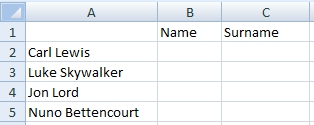Select cells A2:A5 and go to the Text to Columns tool. You can find it on the menu Data.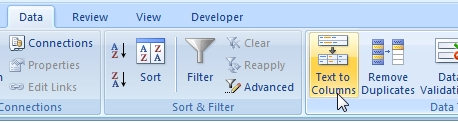Excel show you the Wizard, which helps you. In the Step 1 select Delimited.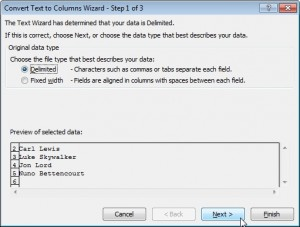Step 2 is to determine the delimiters. In this example is delimiter Space.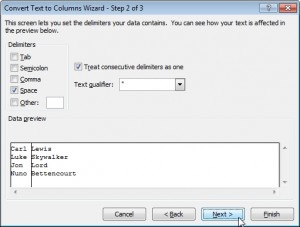In the Step 3 just select Destination, where do you want to place divided values. I choose cell B2.

Tip: Try choose cell A2.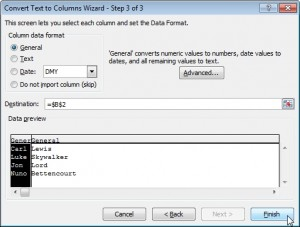This is the result.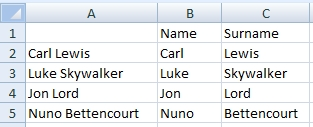## Example 2

In this example is the delimiter a comma. In the first column of the table is information about company. Let’s split it into the separate columns.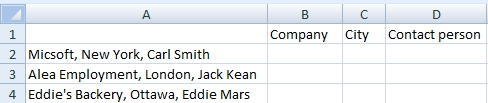We will use the Text to Columns again. Steps will be nearly the same as in the first example. Only in the Step 2 will be the delimiter Comma.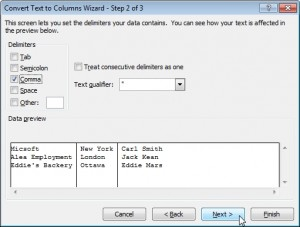And this is the result.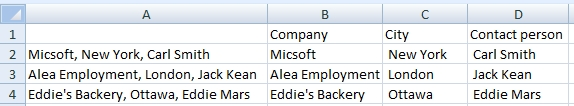## Example 3

Now we will divide the value by using Excel functions. The following values have to be split to Zip code and City. We assume that the postcode is always 5 characters long (various lengths is shown in the next example).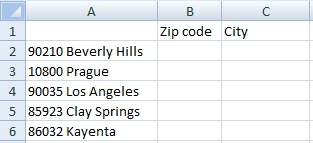Formula in the cell B2:

=LEFT(A2,5)

Function LEFT takes the first 5 characters of the value.

Formula in the cell C2:

=RIGHT(A2,LEN(A2)-6)

Function RIGHT takes the last characters of the value. Formula LEN(A2)-6 calculates how many characters it will be. Function LEN returns number of characters in the value. Zip code + space=6 characters which are not to be displayed from the beginning of the value.

The result: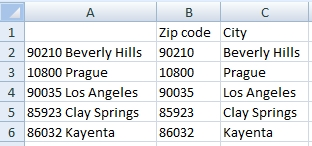## Example 4

Here is a more difficult task. The following values have to be split to Zip code and City. But number of characters in Zip code is various.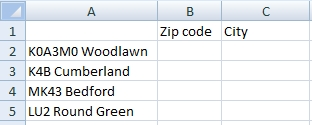Formula in the cell B2:

=LEFT(A2,FIND(” “,A2)-1)

Function LEFT returns the first characters before the space. Function FIND returns position of the first space in the value. We have to subtract 1, because the result value should not contain a space at the end.

Formula in the cell C2:

=RIGHT(A2,LEN(A2)-FIND(” “,A2))

Function RIGHT returns the last characters of the value. Formula LEN(A2)-FIND(” “,A2) calculates how many character it will be.

The result: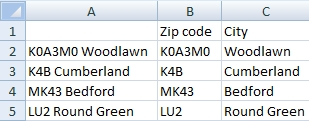Tip: If you do not have values separated by a space, but for example by a comma, modify the formula as follows:

=LEFT(A2,FIND(“,”,A2)-1)

=RIGHT(A2,LEN(A2)-FIND(“,”,A2))